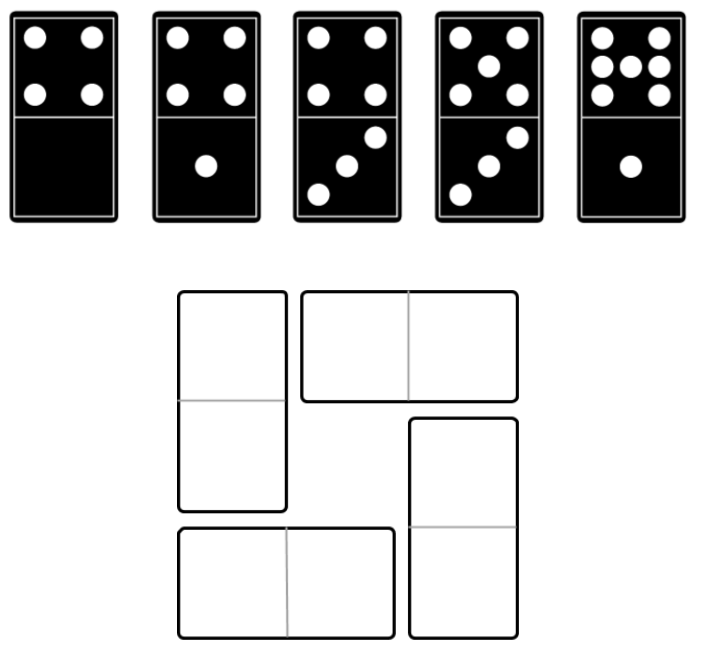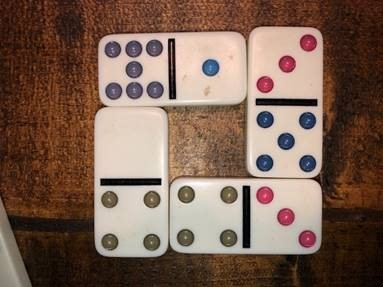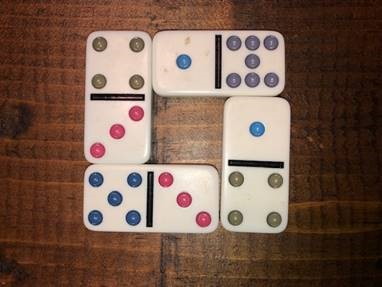Home > Grade 1 > Domino Window 1

# Domino Window 1

Directions: Use four of these dominoes to form a square with the same number of dots on each side.### Hint

What is the greatest sum you can make?
What is the least (smallest) sum you can make?

There are many possibilities. One possibility is the sum of each side is 11.Another solution is the sum of each side is 12.Source: Joshua Nelson

## Open Number Line

Directions: Use the digits 1 to 9, at most one time each, place a digit …